# The Problem (from last week)

We have a globe. We have sampled 13 points (by spinning the globe around): 6 of these were land and 7 were sea. We want to know what proportion of the globe is land. We call each data point a “trial”.

Because of the way we sampled, we can assume that the probability of each point being Land is constant, and these are independent of each other. From this, we can assume that if we did the same experiment many times, the outcomes would follow a binomial distribution. Figure 1 shows a binomial distribution, i.e. the possible outcomes if we had 13 trials, and the probability of land was 0.4.

$Pr(n = r | N, p) = \frac{N !}{r! (N-r)!} p^r (1-p)^{N-r}$

PrLand <- 0.4
nTrials <- 13
n <- 0:nTrials
Pr <- dbinom(n, nTrials, PrLand)

plot(n, Pr, type="h", lwd=20, lend=3,
xlab="Number of Land observations", ylab="Frequency")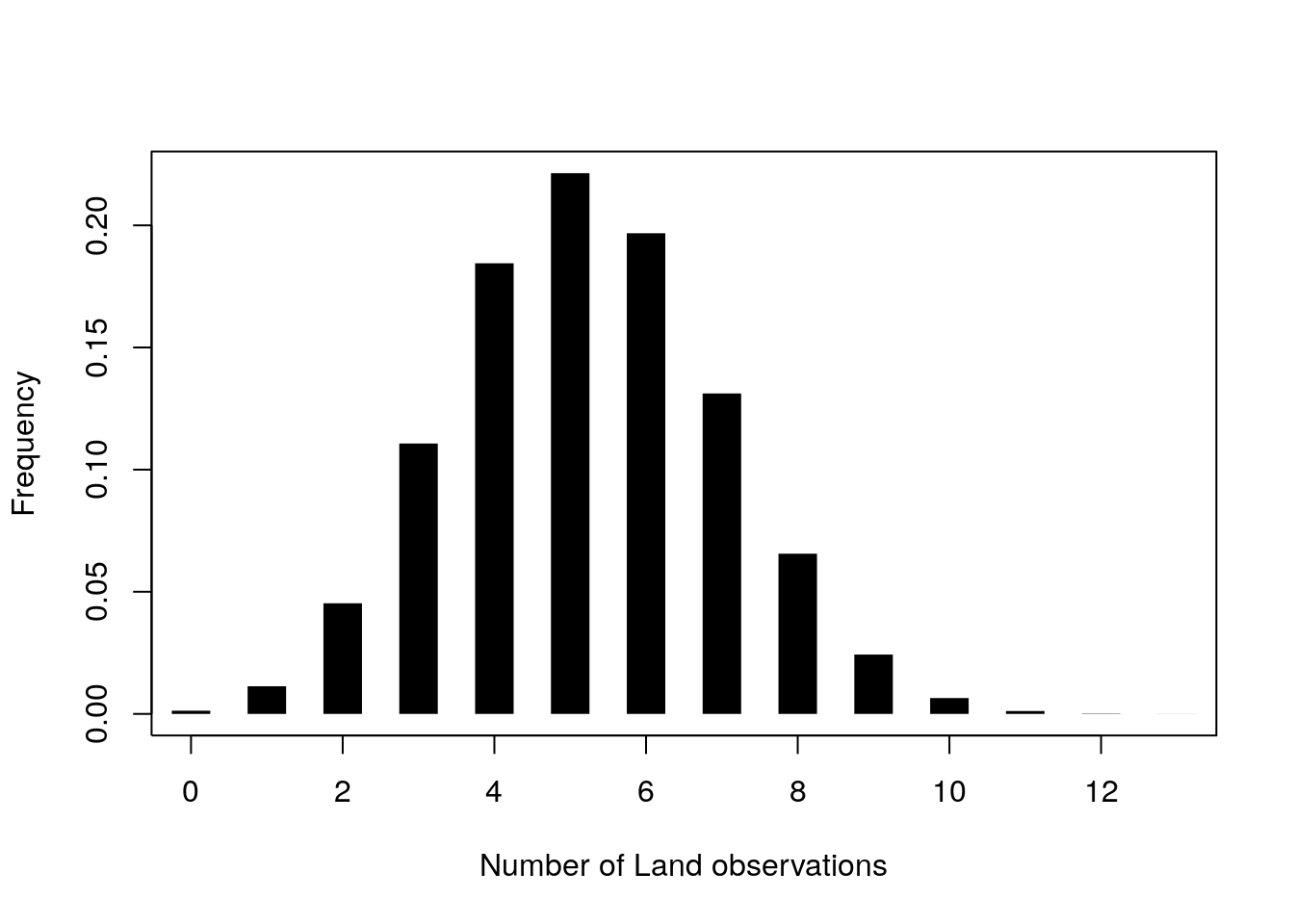Fig. 1: A binomial probability

Fig. 1 is a plot of the probabilities of different outcomes if $$p=0.4$$. But in reality we do not know what $$p$$ is: this is what we want to estimate. If we have some data (e.g. $$n=6$$), we can draw a curve that shows the probability as a function of $$p$$. We have drawn one in Fig. 2. From this we can see that we are more likely to get the data if the probability is close to 0.5, and that values from around 0.3 and 0.6 are also reasonable. But we want to be more precise about this, so we want to (a) find the value that is most likely to give the data, and (b) find some measure of spread around that which suggests what values are likely.

nLand <- 6
p <- seq(0.01, 0.999, length=500)
Pr <- dbinom(nLand, nTrials, p)

plot(p, Pr, type="l",
xlab="Probability of Land", ylab="Likelihood")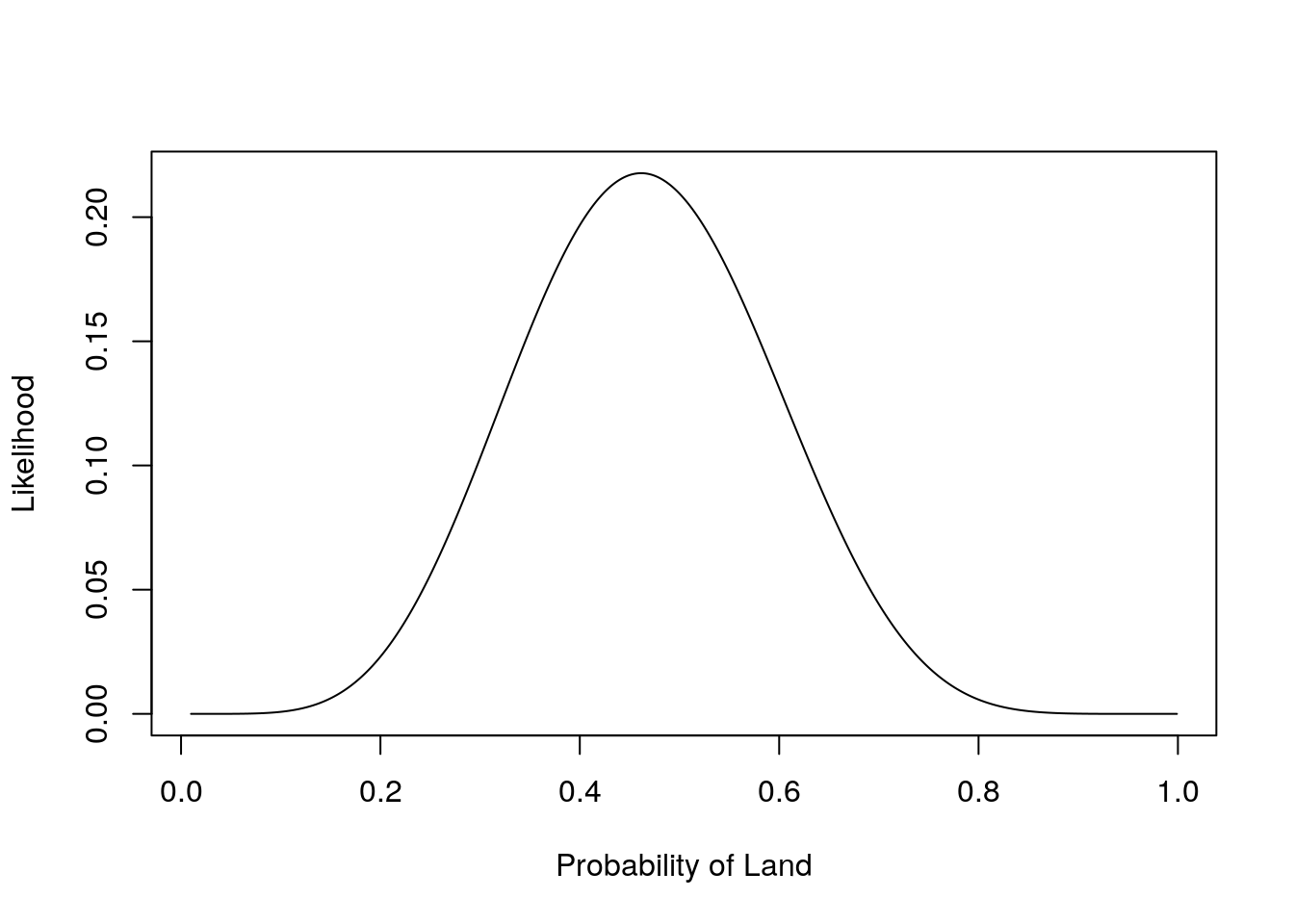Fig. 2: A binomial likelihood

## Finding the MLE

The probability, $$Pr(n = r | N, p)$$, is a mathematical description of how the data came about: it is a statistical model. We assume that there is some unknown “true” value of the parameter, and this is what we are trying to estimate. Thus the parameter is fixed, and the data are what varies. We thus want to find the parameter which is most likely to give rise to the data.

We can vary $$p$$, and calculate $$Pr(n = r | N, p)$$, so that $$Pr(n = r | N, p)$$ is a function of $$p$$. When the probability of the data is a function of the parameters, we call this the likelihood. Our aim is to find the best estimate of $$p$$, i.e. the one which has the highest likelihood of giving the data. We thus want to maximise the likelihood: the estimate we get is called the maximum likelihood estimator, which is often abbreviated to m.l.e., or MLE. There are several ways to find the mle. Here we will find an analytic solution, i.e. use pencil and paper, to calculate the expression for the m.l.e.

## Maximising the likelihood: the maths

The maximum of the likelihood is at the same value of $$p$$ as the maximum of the log-likelihood, and it is easier to woth on the log scale. So we will work with $$\log(L(p|N, r)) = l(p|N, r)$$

$l(p | n) = \log(N !) - \log(r!) - \log((N-r)!) + r \log(p) + (N-r) \log(1-p)$

Becuse this is a function of $$p$$, not $$N$$ or $$r$$, several terms are constants:

$l(p | n) = r \log(p) + (N-r) \log(1-p) + C$

We differentiate with this respect to p to get

$\frac{d l(p | n)}{dp} = \frac{r}{p} - \frac{N-r}{1-p}$

Then we set the gradient to 0, $$0 = \frac{r}{p} - \frac{N-r}{1-p}$$, and rearrange to end up with

$\hat{p} = \frac{r}{N}$

## This Week: Uncertainty in the Estimate

Because different samples give different estimates, we want to quantify this, to suggest plausible values. First, let’s set up the data (from last week), the likelihood and the MLE:

# Source the script directly from the internet
source('https://www.math.ntnu.no/emner/ST2304/2020v/Week02/Week2Functions.R')
nTrials <- 13
nLand <- 6

phat <- mleGlobe(NLand = nLand, NTrials = nTrials)  # The MLE

SimsMLE <- simGlobe(6/13, 13, 1000)["Land",] # simulate data from MLE
Sims6 <- simGlobe(0.6, 13, 1000)["Land",] # simulate data from different estiamte of p

# Plot simulated data, and distribution of estimates of p
par(mfrow=c(1,2)) # set up the plotting window to have 2 panels
# Plot 1 - the distribution of simulated datasets when p = 6/13
h.mle <- hist(SimsMLE, xlab = "Number of land", main = "p = MLE = 6/13", col=1)
# Plot 2 - the distribution of MLE from each of the simulated datasets
hist(mleGlobe(SimsMLE, 13), xlab = expression(hat(p)), breaks=h.mle\$breaks/13,
col=1, main ="Estimate of p")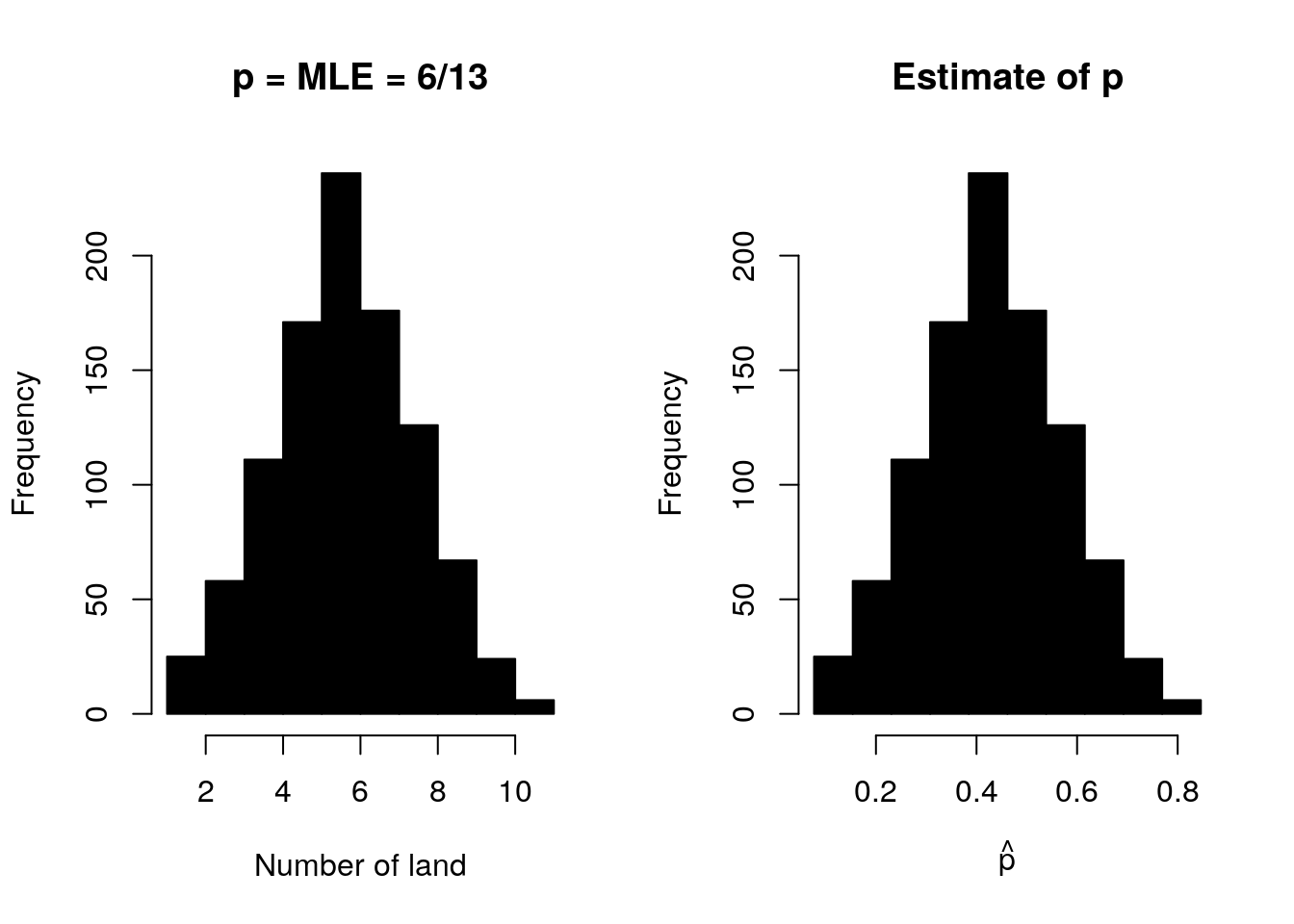We have plotted the likelihood, but we don’t really want to summarise the distibution with a plot: it is easier to use statistical summaries of the distribution. So what statistical summaries could we use?

## Confidence Intervals

Confidence intervals give the range of values of the statistic that are likely. Usually we use 95%.

A 95% confidence interval is an interval that contains the true value in 95% of repeated samples

This is not the easiest definition to understand! We would like to be able to say that a 95% confidence interval has a 95% probability of containing the true value. But unfortunately we cannot say this, because we are assuming the parameter is fixed, and once we have calculated the interval, that is fixed too. So either the interval contains the true value or it does not.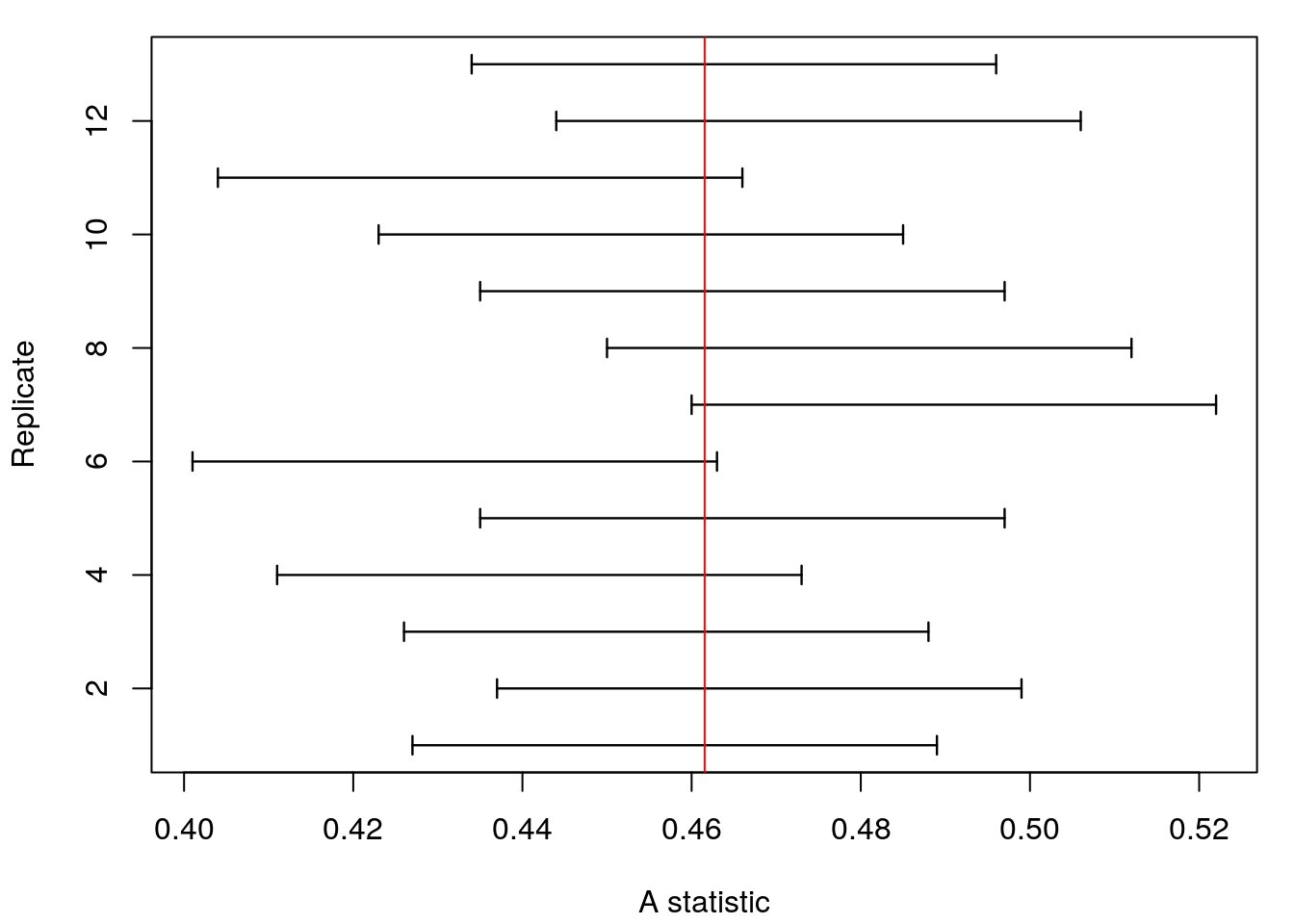Figure: Some confidence intervals, from repeated sampling

But what we can say is that if we took the same sort of sample many times, then 95% of those intervals would contain the true value. The thinking here is that the data are random, and could (in principle, if not practice) be sampled repeatedly. So we are thinking about uncertainty in differnet realisations of the data. By simulating the data many times you are simulating the philosophical idea of repeated samples.

## Constructing a Confidence interval

For continuous data this is straightforward: we exclude the top and bottom 2.5% of the distribution of the statistic.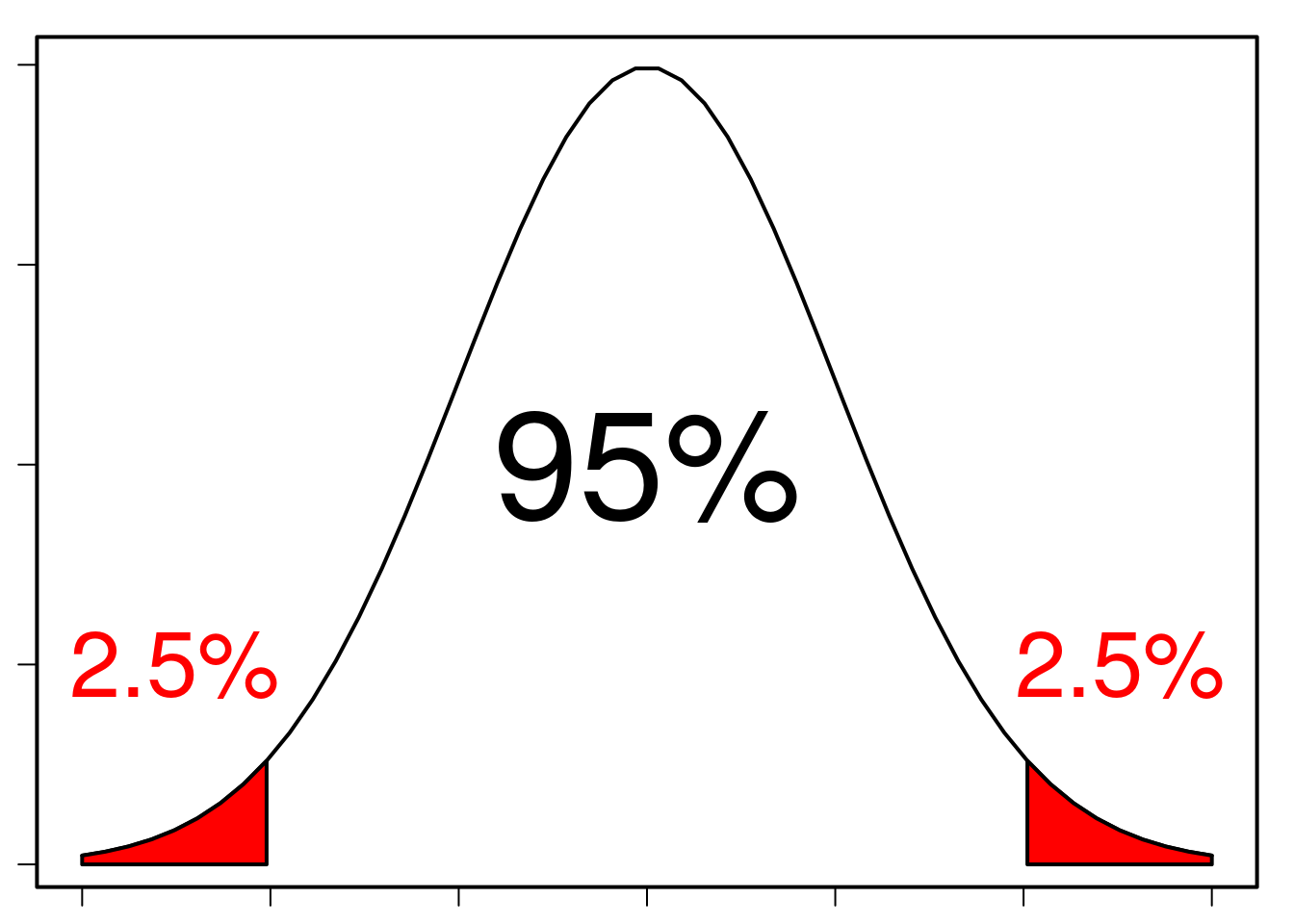For discrete data things are a little more complicated (this is explained below), so we will start by (largely) avoiding the problems and looking at data with a lot of trials. So, imagine we had taken 1000 samples of the (fake) earth, and got 600 Lands out of 1300 trials.

nLandBig <- 600
nTrialsBig <- 1300
pHatBig <- nLandBig/nTrialsBig

The maximum likelihood estimate is 0.46. But what about the confidence interval? Above I explained

[I]f the estimate is correct, then if we randomly sampled the data many times, we would get a distribution of the estimates. We can summarise this distribution by specifying the interval with would contain the estimates 95% of the time.

So, let’s simulate:

For the data estimated above (i.e. with 1300 trials, 600 Land, so p = 0.4615), use simGlobe() to simulate the data you would expect if the MLE was true (see last week’s work, or the use of simGlobe() above). Do 1000 simulations, and plot the distribution of estimates on a histogram

## Confidence Intervals and Quantiles

There are a few ways to calculate confidence intervals. One way is to sort the numbers from lowest to highest

# simulate data (you should do this using the MLE)
SimDist1k <- simGlobe(probability=0.4, NTrials=1e3, nSims = 1e3)["Land",]
# For each simulation, calculate the MLE for that simulated data
SimMLE1k <- mleGlobe(SimDist1k, 1000)
# and sort...
sort(SimMLE1k)[1:10]
##   0.349 0.355 0.355 0.356 0.356 0.358 0.358 0.360 0.362 0.362

and then take the values that are 2.5% of the way from the bottom, and 2.5% of the way from the top:

sort(SimMLE1k)[c(0.025*length(SimMLE1k),
0.975*length(SimMLE1k))]
##  0.368 0.430

The values 2.5% of the way from the bottom, and 2.5% of the way from the top are called quantiles. A x% quantile is a value of a distribution with x% of the distribution less than it

• a median is the 50% quantile
• the 25% and 75% quantiles are called quartiles (in addition to the median, they split the data into 4 quarters)

So, we can just need the 2.5% and 97.5% quantiles. There is a function in R to do this:

quantile(SimMLE1k, c(0.025, 0.975))
##  2.5% 97.5%
## 0.368 0.430

For the data above (600 Lands, 1300 trials), calculate the confidence interval, by simulating data with the MLE, and calculating the quantiles (with the quantile() function).

With simulation there is simulation error: different simulations will give slightly different results, because thesimulations are random. But we know that our data follows a binomial distribution, so we can calculate the probabilities exactly (this would be like having infinite simulations). R, naturally, has a function to do this. Before getting to that function, it is worth remembering the dbinom() function:

r <- 0:10
probs <- dbinom(r, 10, 0.4)
plot(r, probs, type="h", lwd=20, lend=3)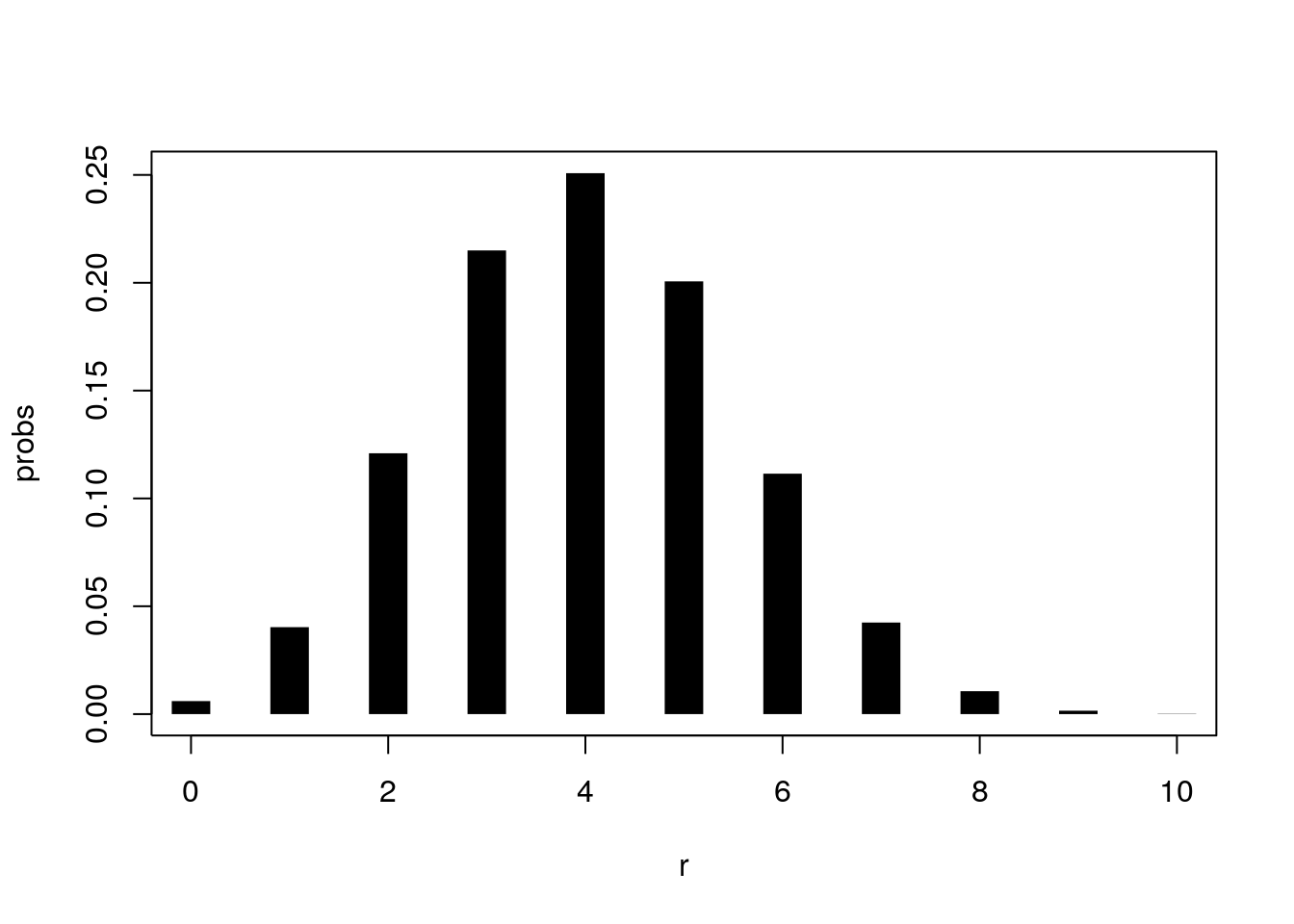This calculates the probability of getting an observation, $$r$$, given the parameters of the distribution (i.e. $$N$$ and $$p$$). But there is also a function called qbinom(), which calculates the quantiles, i.e. the value of r for which $$Pr(n \leq r)=p$$ (e.g. qbinom(c(0.025, 0.975), 10, 0.4)). So we can calculate our confidence intervals with this: use the 2.5% and 97.5% quantiles and the number of trials as the size, and the MLE for the propotion of land as prob:

qbinom(p=c(0.025, 0.975), size=??, prob=??)/??

For the data estimated above, usq qbinom() to calculate the confidence interval. You need to divide by the size, because qbinom() calculates the quantiles for r, and the estimate is r/N. How close is it to the ones you got by simulation?

## What, exactly, is a confidence interval?

Remember, our parameters are fixed, and our data are random. So any statistics we calculate from the data are also random. This means that our confidence interval has to say something about the data (and statistics), not the parameter: the estimator, not the estimand.

A confidence interval is thus an interval that will contain a population parameter (i.e. the true value) a specified proportion of the time. We can look at this by simulating lots of data, calculating the confidence interval for each one, and seeing how many contain the true value:

True <- 0.4
N <- 13
ConfIntervals <- CIGlobe(NLand=SimDist1k[1:20], NTrials=1000)

rep <- 1:nrow(ConfIntervals)

par(mar=c(4.1,4.1,1,1))
plot(1,1, type="n", xlim=range(ConfIntervals), ylim=range(rep), xlab="A statistic", ylab="Replicate")
arrows(x0=ConfIntervals[,"Lower"], y0=rep, x1=ConfIntervals[,"Upper"], y1=rep, lwd=1.2, angle = 90, code=3, length=0.05)
abline(v=True, col=2)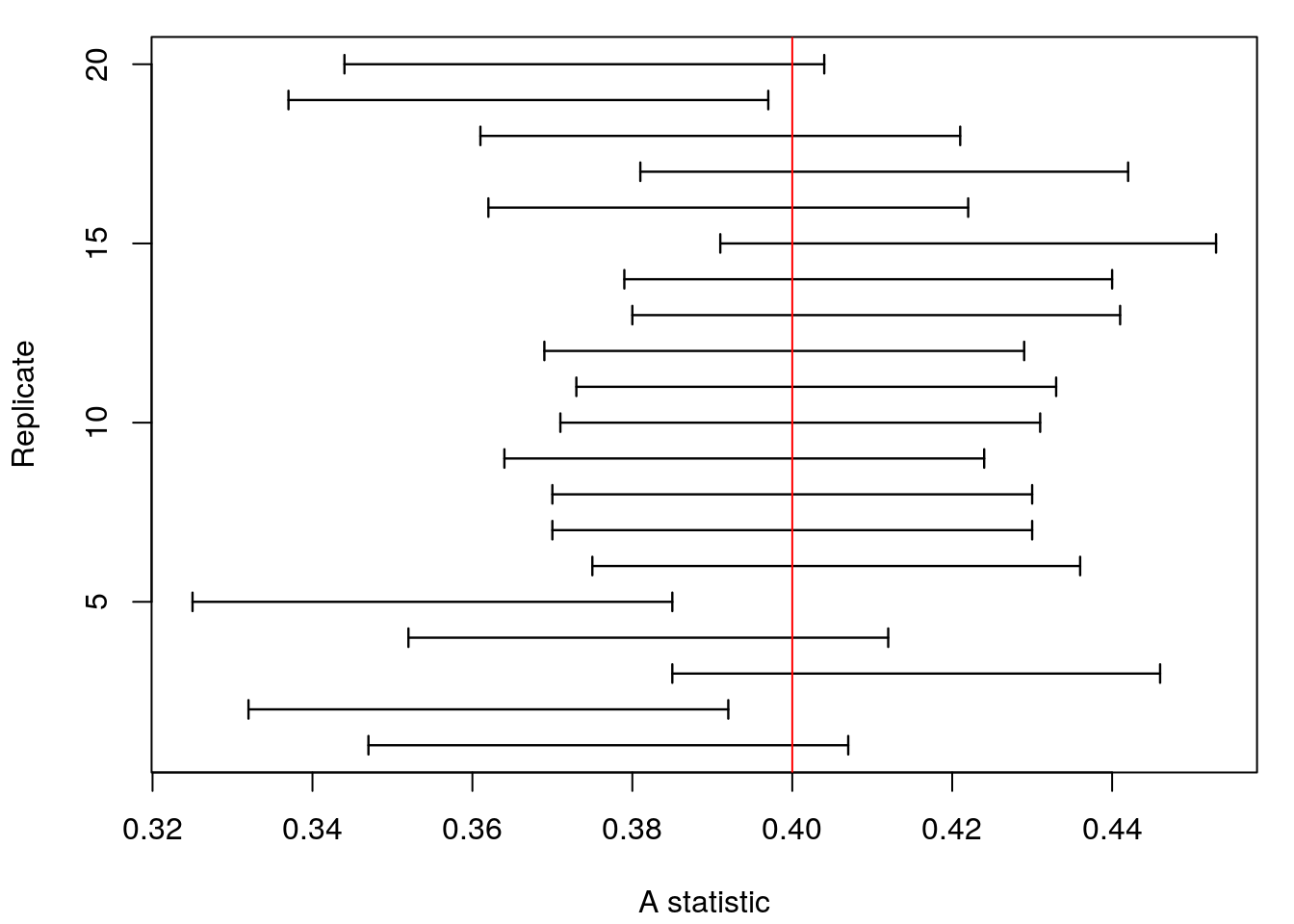# Calculate proportion of CIs containing true value
IsInCI <- function(CIs, True) {
# Check if Lower < True < Upper
InCI <- CIs[,"Lower"] < True & CIs[,"Upper"] > True
# Return proportion where InCI is TRUEmistakes
mean(InCI)
}

IsInCI(ConfIntervals, True = 0.4)
##  0.85

i.e. if we repeatedly sample the same population, 95% of confidence intervals will include the “true” parameter

For the estimate of $$p$$ calcluated above (i.e. from 600 Lands and 700 Seas), simulate the data, calculate the confidence intervals for the data and then estimate the proportion of confidence intervals containing the “true”" value.

## Confidence Interval for a Binomial with differnet Ns

If the data are discrete then the estimate is discrete, e.g. if we have 4 trials the only possible estiamtes are 0, 0.25, 0.5, 0.75 and 1.0. We can’t guarantee that we will get exaclty 95% of the distribution.

Because the data are discrete, we have a smaller number of possible values of the confidence interval: if we have 5 trials (say), the estimates of p can only be 0, 0.2, 0.4, 0.6, 0.8 and 1. So the limits of the confidence interval can only be these values too:

N.small <- 5
p.small <- 3/5 # if we observe 3 successes

qbinom(p=c(0.025, 0.975), size=N.small, prob=0.5)/N.small
##  0 1

Which is annoying. What it means is that a 95% confidence interval might not contain the data 95% of the time. Technically, the actual proportion contained by a confidence interval is called the coverage. So, in the example above the coverage is 100%, not 95%. This is not good. But it improves as the sample size increases, so is only really a problem when you don’t have enough data to say anything sensible.

For the original data (i.e. 6 Lands and 7 Seas), calculate the confidence interval. How does it compare to the 600 Land, 700 Seas? THen do this for 60 Land and 70 Seas.

## Approximations

When $$N$$ is large, everything becomes easier (this is a general rule in statistics). As $$N$$ gets larger, The likelihood looks more and more like a normal distribution (this is also a general rule in statistics). So, we can calculate the confidence interval by assuming the likelihood is a normal distribution. Although the advantage isn’t obvious in this case, the same approach works for a lot of problems, thanks to the genral rule that likelihoods look more and more like a normal distribution when the sample size increases.

So, if the likelihood is approximately normal, what normal distribution is it approximate to? In other words, what are the mean and standard deviation of the normal distribution? The mean is straightforward: it is the maximum likelihood estimate, i.e. $$\hat{p}=r/N$$. And the standard deviation is simply the standard error, $$\sqrt{\hat{p}(1-\hat{p})/N}$$. So,

$l(p|N, n) \sim N(\hat{p}, \sqrt{\hat{p}(1-\hat{p})/N})$

We can use this approximation to find the 95% confidence interval. For a standard normal distibution (i.e. a normal with mean 0, variance 1), the interval is (-1.96, 1.96). So for a binomial, we can calculate the interval by transforming the standard normal:

$\left( \hat{p} - 1.96 \sqrt{\hat{p}(1-\hat{p})/N}, \hat{p} + 1.96 \sqrt{\hat{p}(1-\hat{p})/N} \right)$

So, for $$N=13$$, $$n=6$$ (Land) the interval is (0.4 - 0.14, 0.4 + 0.14), i.e. (0.26, 0.54).

This is easy to calculate in R:

# This is NOT from above
NSuccess <- 400
nTrials <- 1000
pHat <- NSuccess/nTrials

# Calculate standard error
#  This could be done in 1 line, but in 2 lines makes it easier to read
Var <- pHat*(1-pHat)/nTrials
StdError <- sqrt(Var)

(ConfInt <- c(pHat - 1.96*StdError, pHat + 1.96*StdError))
##  0.3696358 0.4303642

We also have a function to do this:

AssymptoticCIGlobe(NLand=400, NTrials=1000)
##     Lower     Upper
## 0.3696358 0.4303642

For the data above (600 lands, 700 Seas), calculate the approximate confidence interval.

## How well are we doing?

We should check the coverage of the approximation.

Check the coverage of the approximation. Use the code from the “What, exactly, is a confidence interval?” section: calculate confidence intervals from simulated data with CIGlobe(), and with AssymptoticCIGlobe(). Then use the IsInCI() function to check to see if each simulated confidence interval contains the true value, and calcluate the proportion that do.

## Standard Errors

So far we have been looking at confidence intervals. These are helpful for saying what the likely values are. In order to get the approximate confidence interval, we have to calculate the standard error. So, if we want to summarise the uncertainty in the estimate in a single parameter, we could use this statistic. We have already used it above, to calculate the assymptotic confidence intervals, but now we can look at it on its own.

Standard Deviations of Statistics: $$s$$

Binomial variance of $$n$$: $$Var(n|N, p) =Np(1-p)$$

Our statistic: $$n/N$$

$$Var(n/N) = 1/N^2 Var(n) = p(1-p)/N$$

Standard error:

$$s = \sqrt{p(1-p)/N}$$

Calculate the standard errors for data of different sizes (e.g. 6 land and 7 seas, 60 land and 70 seas, and 600 land and 700 seas): how does the standard error change with the sample size? Either write your own code, or use the StdErrGlobe(´`) function (e.g.StdErrGlobe(NLand=400, NTrials=1000))